﻿ Extreme Values of Functions (Max/Min)

#Extreme Values of Functions (Max/Min)

Roughly, ‘extreme values’ are the $y$-values of high/low points on a graph.

You also usually want to know the $x$-value(s) where these high/low points occur.

The $y$-value of a highest point is called a maximum value.

The $y$-value of a lowest point is called a minimum value.

As illustrated below, the ONLY places that extreme values can occur are at horizontal tangent lines, kinks/breaks, and endpoints.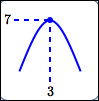maximum value is $\,7\,$; it occurs at $\,x = 3\,$minimum value is $\,2\,$; it occurs at $\,x = 3\,$

The plural of maximum is maxima.

The plural of minimum is minima.

Maxima/minima can occur at horizontal tangent lines.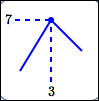maximum value is $\,7\,$; it occurs at $\,x = 3\,$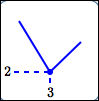minimum value is $\,2\,$; it occurs at $\,x = 3\,$

A ‘kink’ is a sudden change in direction.

Maxima/minima can occur at kinks.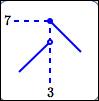maximum value is $\,7\,$; it occurs at $\,x = 3\,$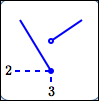minimum value is $\,2\,$; it occurs at $\,x = 3\,$

Imagine tracing a graph with a pencil.

A ‘break’ is a place where you have to pick up the pencil.

Maxima/minima can occur at breaks.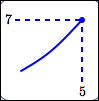maximum value is $\,7\,$; it occurs at $\,x = 5\,$minimum value is $\,2\,$; it occurs at $\,x = 1\,$

Maxima/minima can occur at endpoints.

The only places that extreme values can occur are at horizontal tangent lines, kinks/breaks, and endpoints.

But, you don't have to have an extreme value at such places!

In all the examples below, there are greater and lesser values arbitrarily close by.Horizontal tangent line:
no extreme value hereKink:
no extreme value hereBreak:
no extreme value here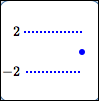Endpoint:
no extreme value here

To the left of the endpoint, the function oscillates: it's $\,2\,$ for rational inputs, and $\,-2\,$ for irrational inputs.

You have to work hard to get an example of an endpoint that's not a max or min!

## A Discussion of Extreme Values from a Non-Graphical Viewpoint

Suppose you have a function $\,f\,.$ A function is a rule—it takes an input, does something to it, and gives a corresponding output. The function $\,f\,$ can be viewed as a box:• Drop an input $\,x\,$ in the top
• $f\,$ does something to the input
• The corresponding output $\,f(x)\,$ drops out the bottom

For extreme value questions, you have the following scenario:

You're interested in a particular set of inputsit might be the entire domain; it might be some interval.

You drop these inputs of interest into the function box, and get a corresponding pile of outputs.

Take a look at this output pile:• Is there a greatest number in the output pile?

That is, can you pick up a number that is greater than or equal to all the other numbers in the pile?

If so, we call this number an extreme value—in this case, a maximum.

• Is there a least number in the output pile?

That is, can you pick up a number that is less than or equal to all the other numbers in the pile?

If so, we call this number an extreme value—in this case, a minimum.

In both cases, we likely want to know what input(s) gave rise to these ‘extreme’ outputs.

Extreme value questions have to do with finding the greatest/least values of the output of a function, and figuring out what input(s) they came from.

## Greatest/Least Overall?Or Just Close By?(Global versus Local Max/Min)

Sometimes you're interested in highest/lowest values overall (like at B, E, and F). These are called global (or absolute) max/min.Sometimes you're interested in ‘local’ highest/lowest values (like at A, C, and D). These are called local (or relative) max/min. These are highest/lowest, provided you don't look too far away!

[Note: You'll learn in Calculus that a global max is also a local max, and a global min is also a local min.]

Some people like the terminology global/local. Others like absolute/relative. You choose!

Calculus provides language and tools to enable precise discussions of extreme values and related concepts. The informal language used here (e.g., ‘high/low points’, ‘breaks’, ‘kinks’) will all be made precise in Calculus. This section is only an informal introduction.

The field of Operations Research is devoted to maximizing or minimizing real-world objectives. Maximize profits. Minimize costs. Minimize the amount of material needed to construct a box with a specified volume. Extreme values have extreme applicability!

## And Finally—You May Not Have Extreme Values!

You may not have any extreme values. You might have a max, but not a min. You might have a min, but not a max.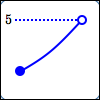In the graph shown, the number $\,5\,$ is ‘trying to be’ the maximum value. But, there's no $\,5\,$ in the output pile, because the point isn't there!

For example, you could pick up a $\,4.9\,$ from the output pile—but this wouldn't be greatest, because there's a $\,4.99\,$ there!

And $\,4.99\,$ isn't greatest, because there's a $\,4.999\,$ there (and so on).

There's an important theorem in Calculus, called the Max/Min Theorem (or the Extreme Value Theorem) that guarantees conditions under which you'll have both a max and a min. Calculus is so wonderful!